# ENGI 3423 - Normal Probability Plot Simulation using MINITAB

In this session we shall use Minitab® to
•   create a simulated set of data for use in Minitab,
•   use Minitab to display a graphical summary of the data,
•   use Minitab to produce a normal probability plot for the data,
•   use Excel® to produce a normal probability plot for the data.

# Creation of a Data Set

 We shall simulate the creation of a normal probability plot for a case where the data are known to be non-normal, namely data drawn from an exponential distribution.   The standard exponential distribution (λ = 1) is shown here: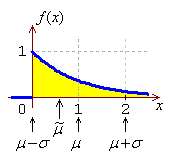Start Minitab. Click on the menu item "`Calc`" Move to "`Random Data`" On the new pop-up menu, click on "`Exponential...`"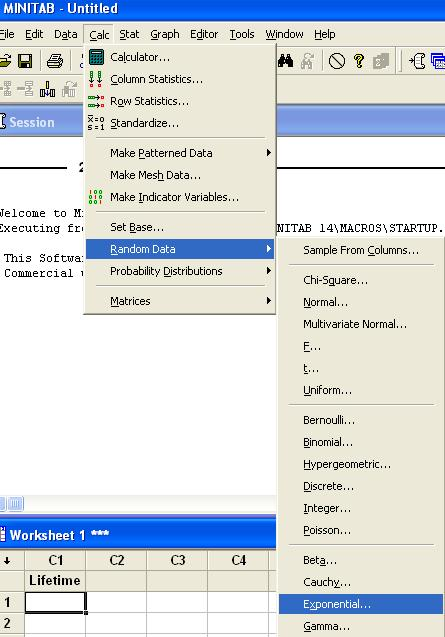In the new dialog box, Enter ` 100 ` for the number of rows, Type ` C1 ` for the location, Enter ` 1000 ` for the Scale, Leave the Threshold at 0 and Click on the "`OK`" button.

 Type a new name for column ` C1 `. As lifetimes are often random quantities that follow an exponential distribution, we shall use the name ` Lifetime ` here. The column ` Lifetime ` now contains 100 values. Note that, as this is a set of random data, the numbers in your data column will not be identical to those shown here.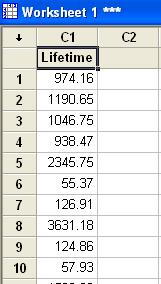# Graphical Summary of the DataAs we have done before, we can use Minitab to gain a first impression of the distribution of our newly-generated data. Click on the menu item "`Stat`", Move to "`Basic Statistics`" and Click on "```Display Descriptive Statistics...```".

 In the dialog box, Double click on "`C1 Lifetime`" to select it into the "`Variables`" pane, then Click on the "`Graphs...`" button.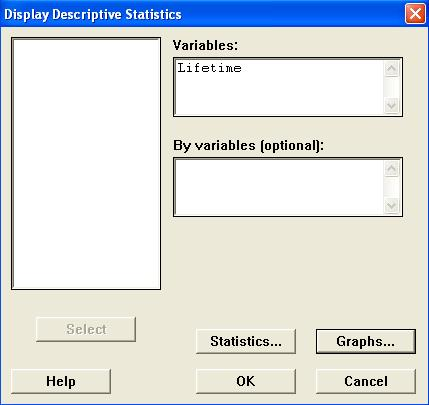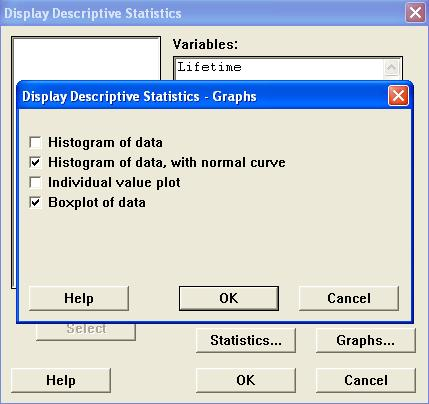In the new dialog box, Check the boxes "```Histogram of data, with normal curve```" and "`Boxplot of data`" and Click on the "`OK`" button. Back in the "```Display Descriptive Statistics```" window, click on the "`OK`" button.

Two graph windows then appear:The evidence for positive skew is overwhelming.
The histogram shows a long right tail and no left tail.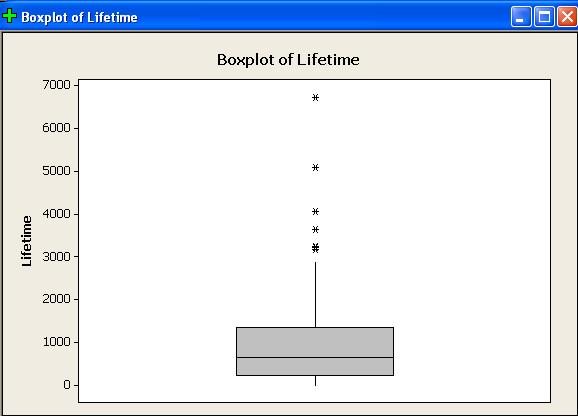The boxplot shows the upper quartile to be further away from the median than the lower quartile.
The boxplot’s upper whisker is much longer than its lower whisker.
There are several outliers, all on the positive side.
From the Session window,The mean is much greater than the median.

[Note also that the values of sample mean and sample standard deviation are consistent with equality of the population mean and population standard deviation, which is true of any exponential distribution.]

Clearly the normal distribution does not fit these data.

# Normal Probability Plot — Minitab

 Click on the menu item "`Graph`", then Click on "`Probability Plot...`"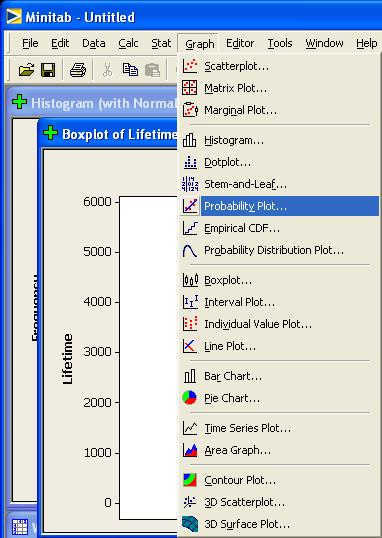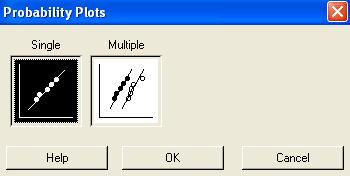Accept the default "Single" Just click on the "`OK`" button.

In the dialog box,

Double click on "`C1 Lifetime`" to select it into the "`Variables`" pane, thenClick on the "`Labels...`" button.

Provide a more meaningful title for the normal probability plot.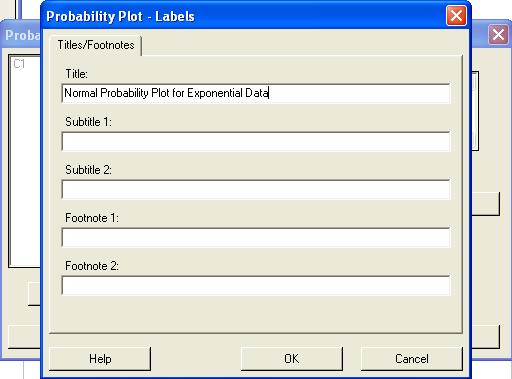Then click on the "`OK`" button.
Back in the "`Probability Plot`" window, click on the "`OK`" button.

The following normal probability plot (or something very like it) then appears: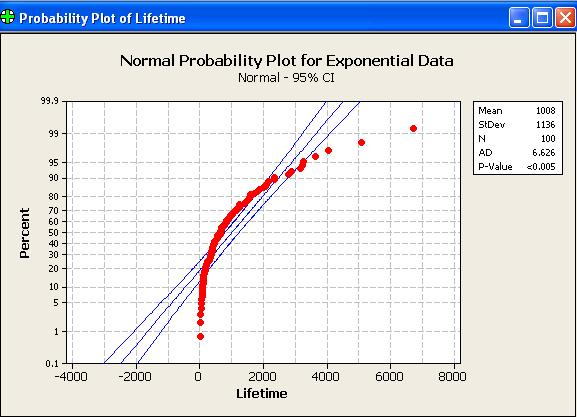A random sample of size 100, drawn from a normal distribution, will have all (or nearly all) of its points near the straight line of a normal probability plot.   Only 5% of all points will fall, by chance, outside the two curves on either side of the line.
Clearly this data set has not been drawn from a normal distribution.

Here are some other normal probability plots (as produced by Version 13.2 of Minitab - mostly the same as Version 15.1).

100 data drawn from a Normal distribution N(1000, 102)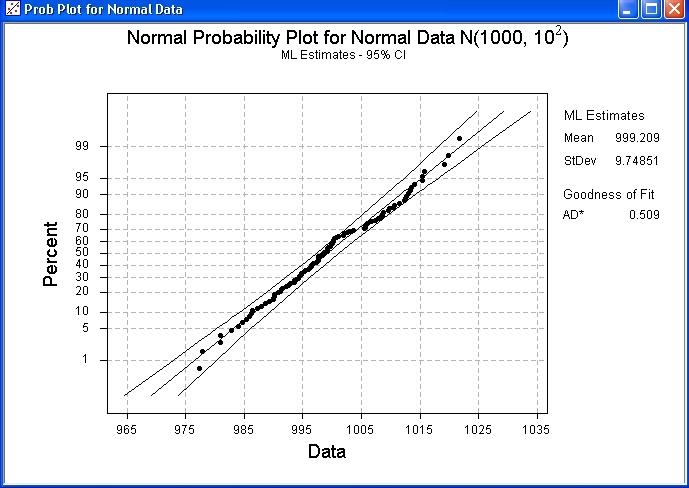Note how nearly all points lie within the two curves, close to a straight line.

100 data drawn from a standard LogNormal distribution.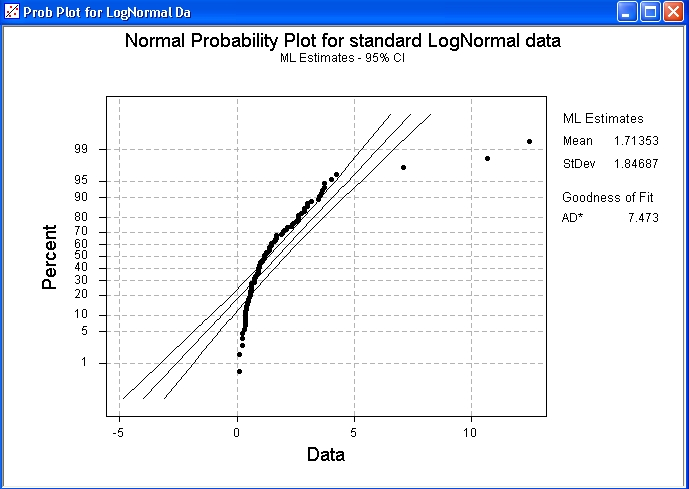Note how this plot resembles that for the exponential distribution.

100 data drawn from a Cauchy distribution of mean 0 and semi-interquartile range 1.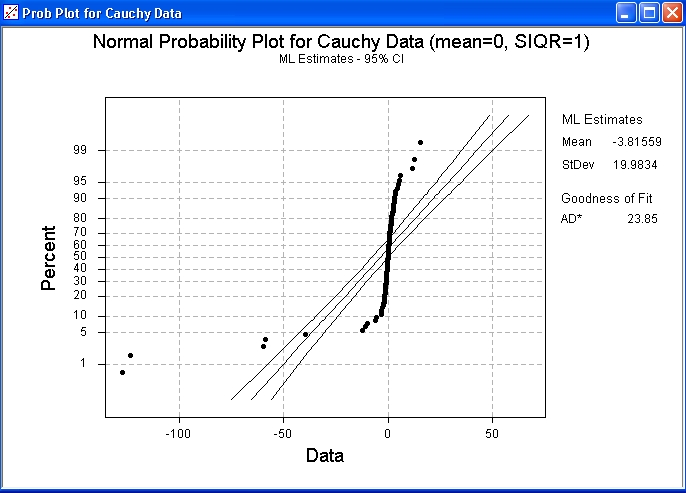This plot reveals the very heavy tails of the Cauchy distribution.
For the run that produced this plot, the 100 data values had a sample median very close to the population median value of zero and quartiles near ±1.   However, the sample mean was below –3.   Recall from Problem Set 5 Question 4 that the Cauchy distribution has a finite interquartile range but an infinite population variance.

100 data drawn from a Beta distribution Beta(18, 2).Note how this plot reveals a strong negative skew, together with the light right tail.   The plot clearly shows that these data are inconsistent with a normal distribution.

You can copy and paste the above results into your Report Pad.
Then save your work and exit from Minitab.

The populations from which these data sets came are illustrated here, in their standard forms.
standard exponentialstandard normal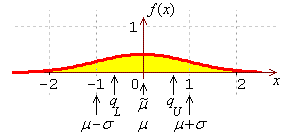standard log-normal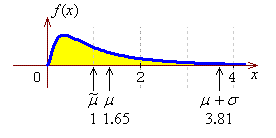standard Cauchybeta (18, 2)# Normal Probability Plot — Excel

Open Excel and import the data set, for which you wish to create a normal probability plot, into a column.

 Check that the Analysis Tool Pack is present, as follows. Click on the menu item "`Tools`" and check if the item "`Data Analysis...`" is present in that drop down list. If it is present, then bypass the next step. Otherwise, click on "`Add-Ins...`".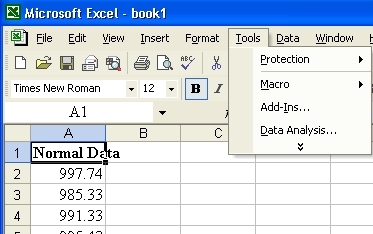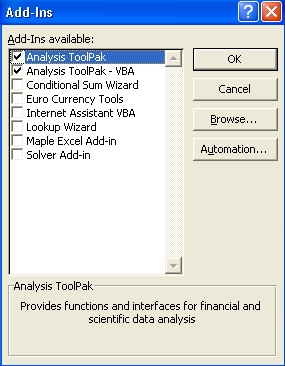Check the options for "`Analysis ToolPak`" and "`Analysis ToolPak - VBA`" then click on the "`OK`" button.

 Alongside the genuine data, place a column containing the same number of values. The new values can be any set of distinct numbers. They are needed for the next two steps.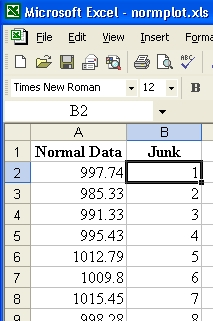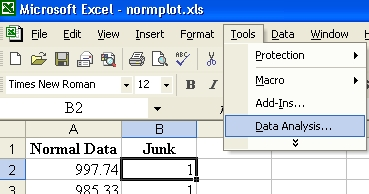Click on the menu item "`Tools`" and then Click on "```Data Analysis...```".

 Scroll down the list of Analysis Tools and click on "`Regression`" then click on the "`OK`" button.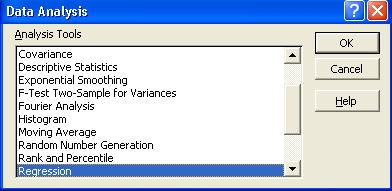In the Regression dialog box: Select the range where your data are stored as the "`Input Y Range`". Select the range where the junk data are stored as the "`Input X Range`". Choose a location for the tables of values, (most of which will be irrelevant!).   In this example, a separate tab has been chosen. Check the last box "```Normal Probability Plots```" and leave all other boxes unchecked. then click on the "`OK`" button.

At the location where the output was chosen to be, various tables and a graph appear.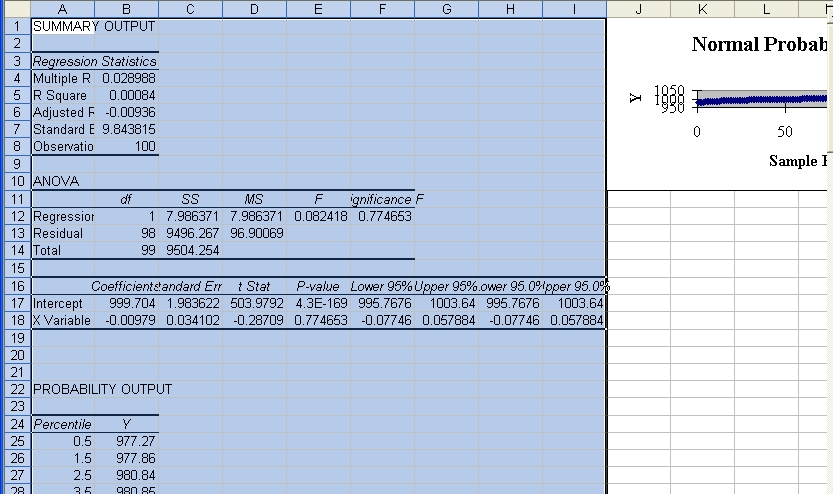Resize the graph and move it to another tab.
Right-click on any of the plotted points.Click on "`Add Trendline...`".
In the new dialog box, accept the default option (linear trend) and
click on the "`OK`" button.
You may also wish to tidy the graph up somewhat, to produce a result like this:The Excel file is available here.[Return to the index of demonstration files]         [To the next tutorial]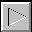[Return to your previous page]

Created 2003 10 19 and most recently modified 2007 07 09 by Dr. G.H. George